# Cyclogenesis Comic, Page 9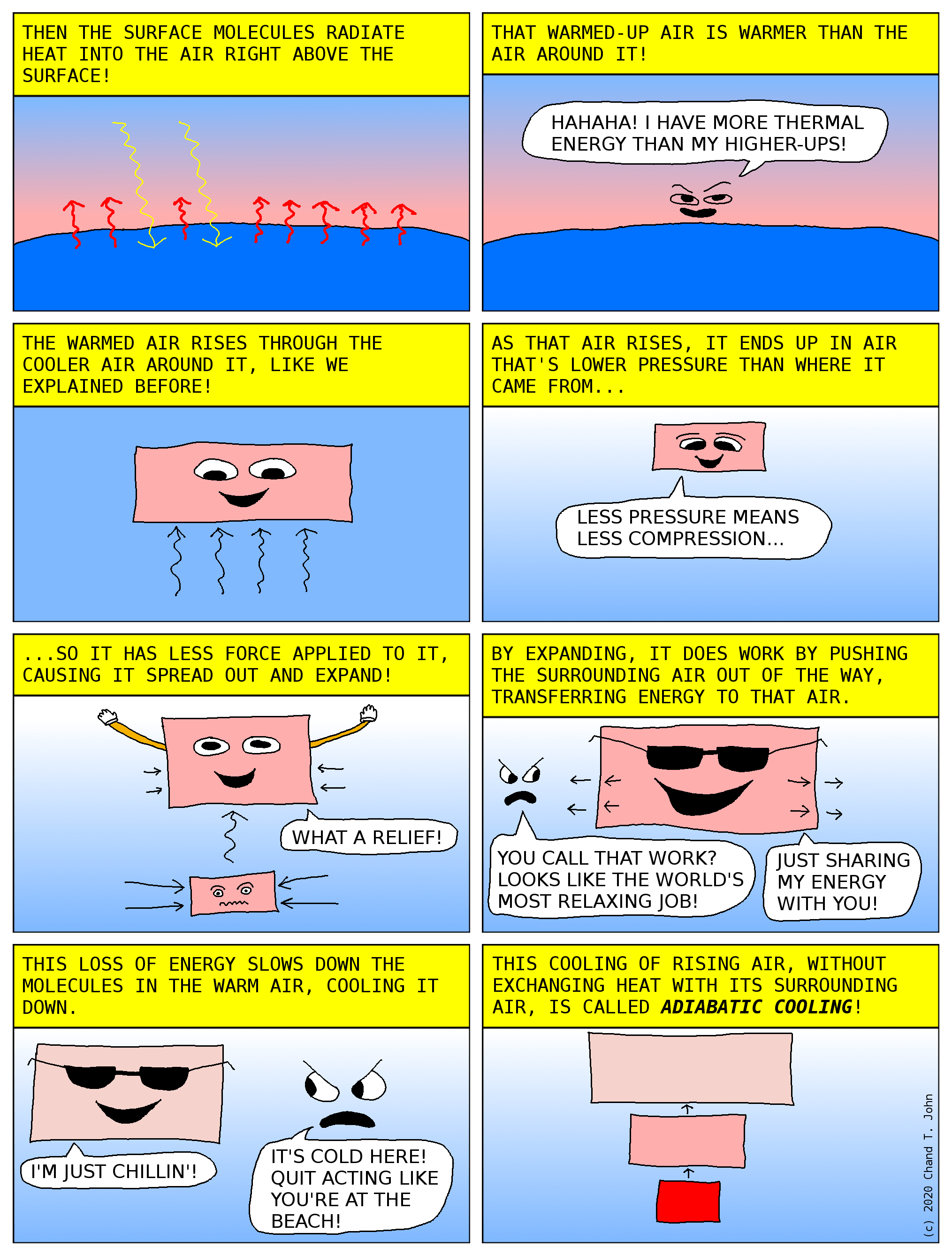# Cyclogenesis Comic, Page 8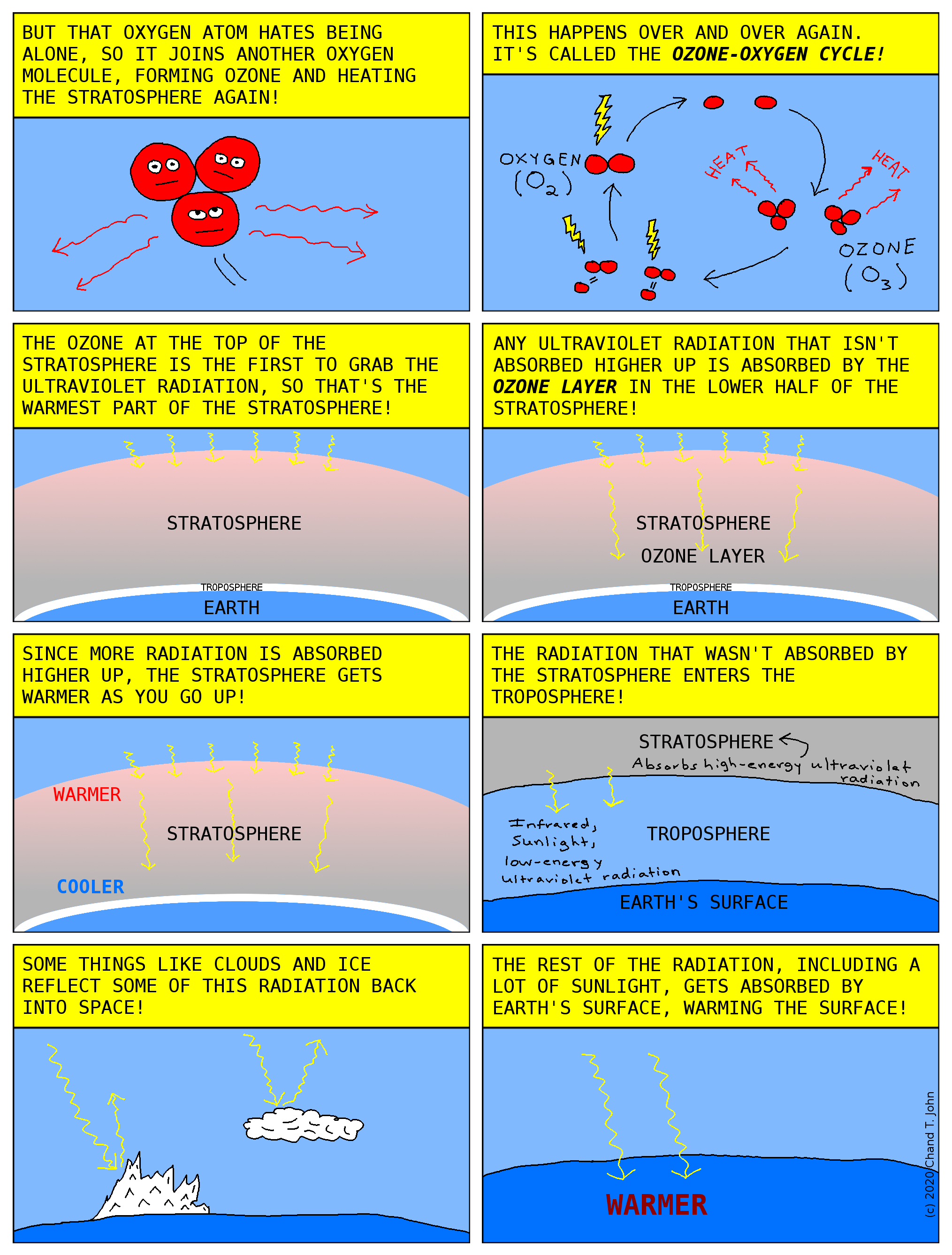# Cyclogenesis Comic, Page 7# Cyclogenesis Comic, Page 6# Cyclogenesis Comic, Page 5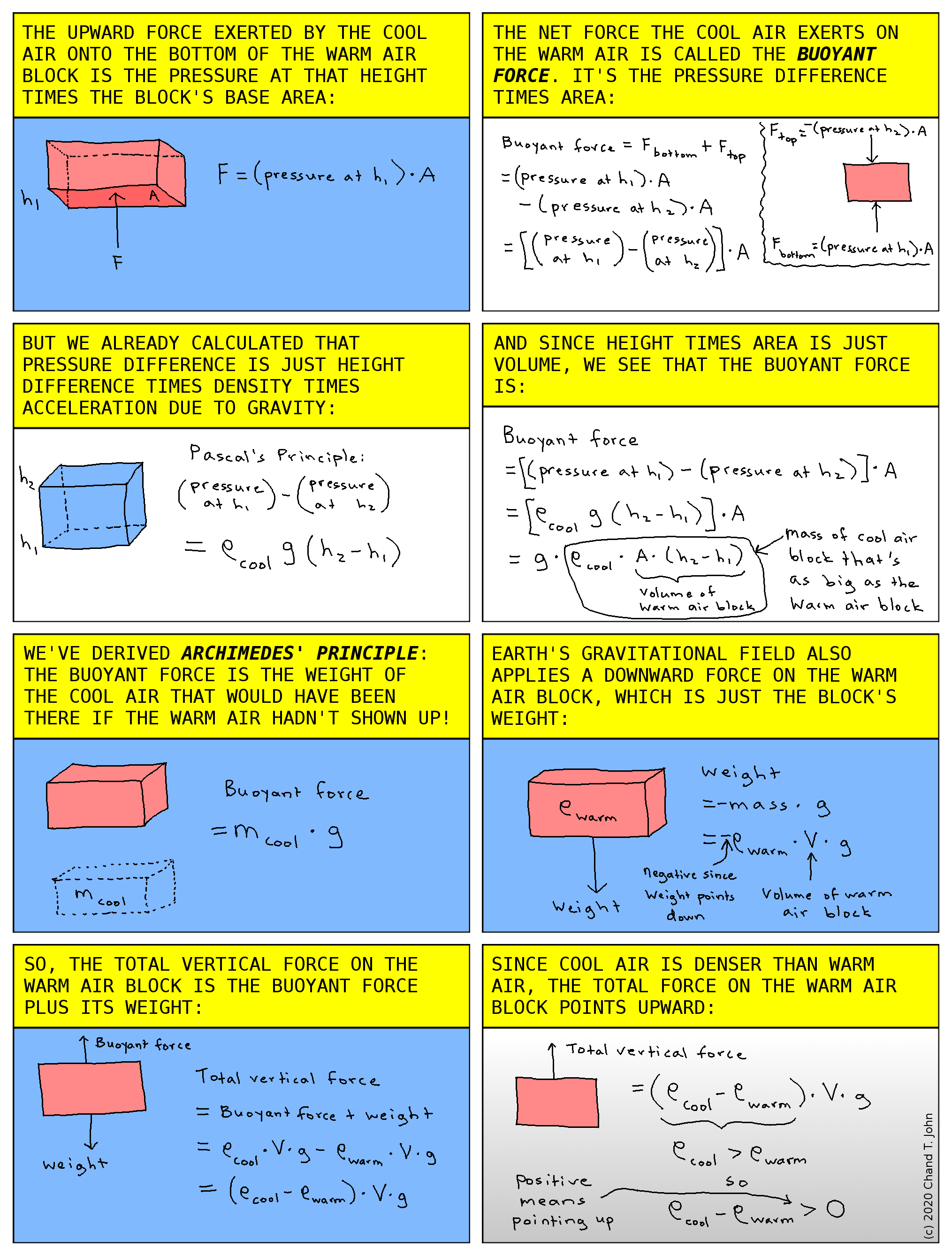# Cyclogenesis Comic, Page 4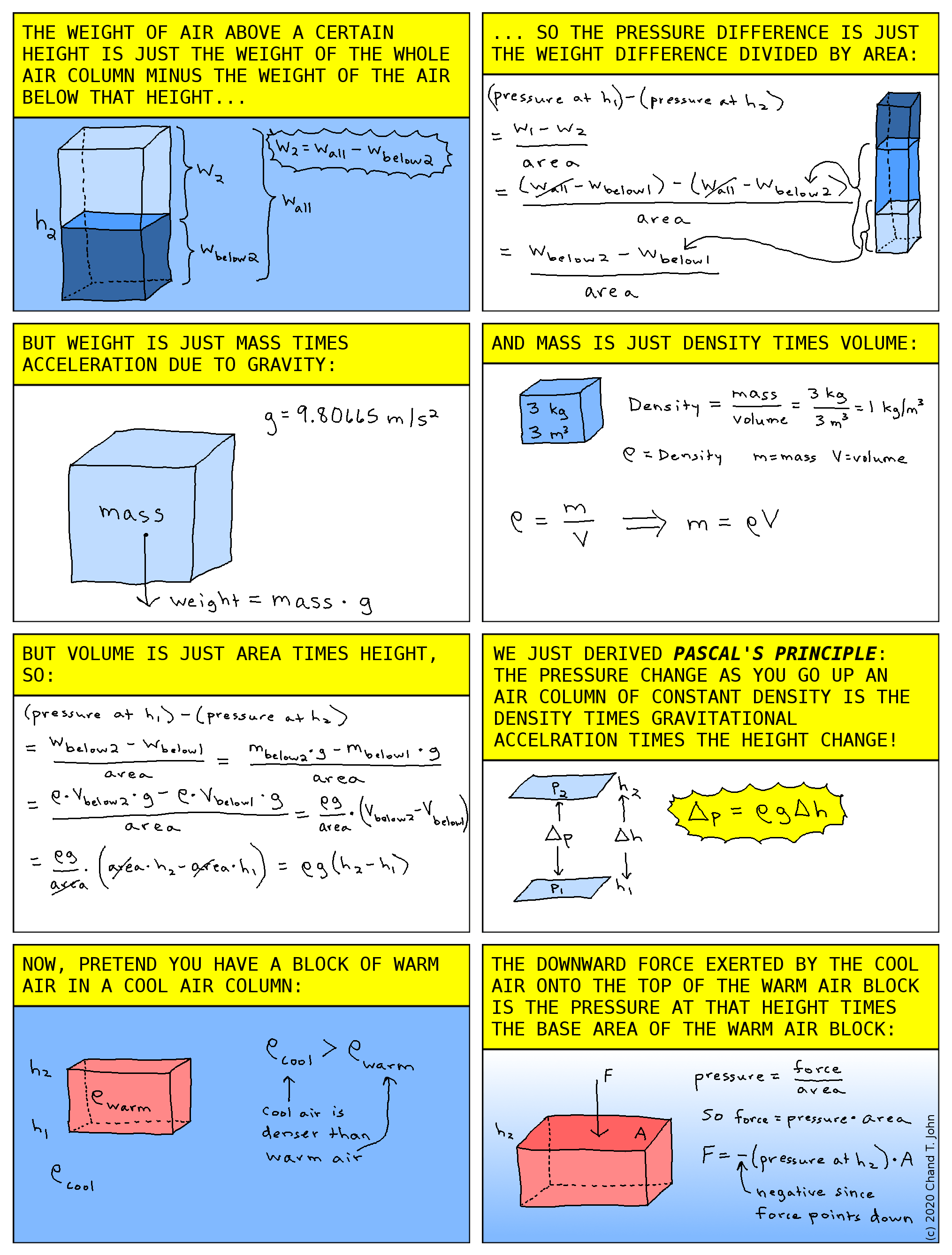# Cyclogenesis Comic, Page 3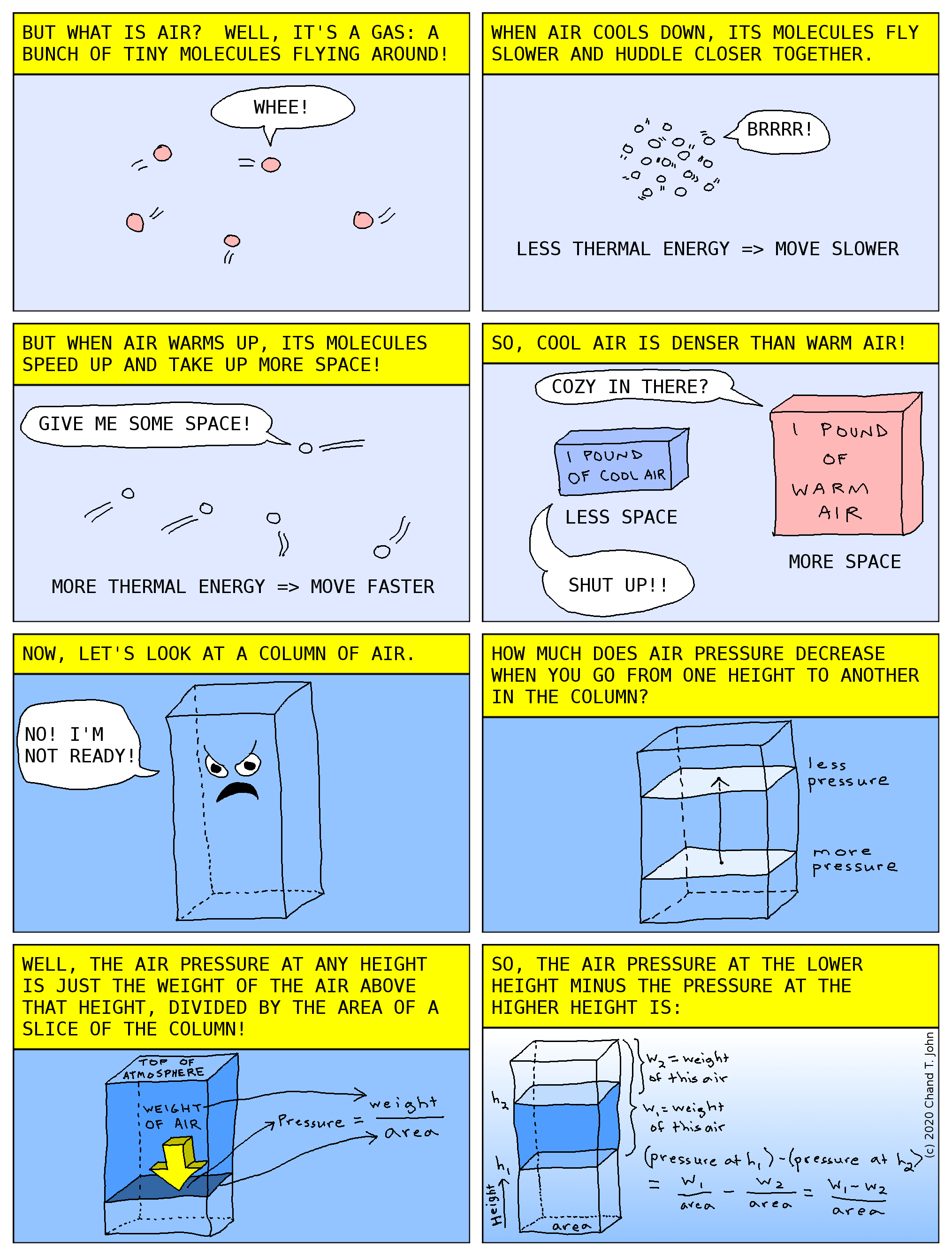# Cyclogenesis Comic, Page 2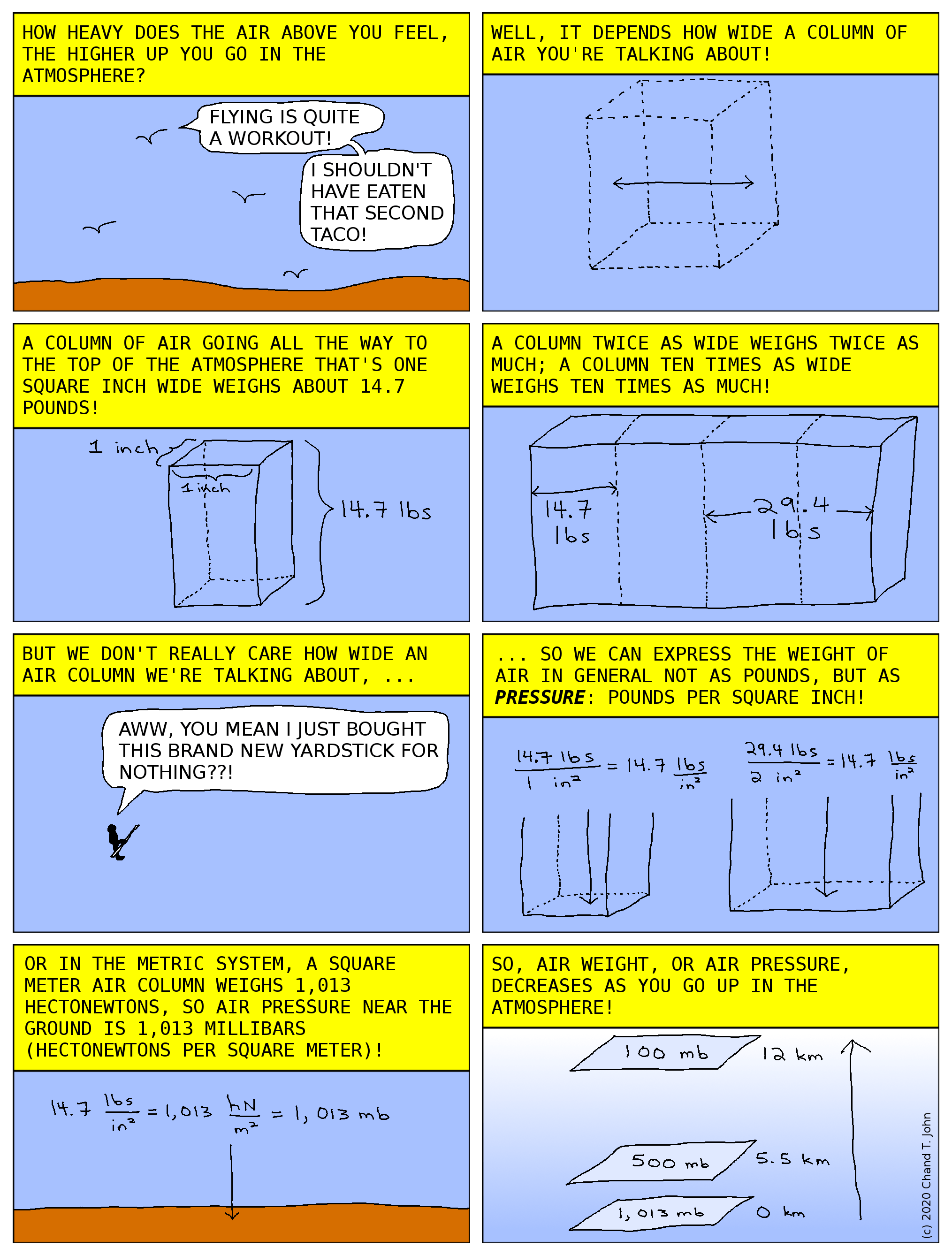# Cyclogenesis Comic, Page 1# Linear Regression & Gradient Descent, Page 9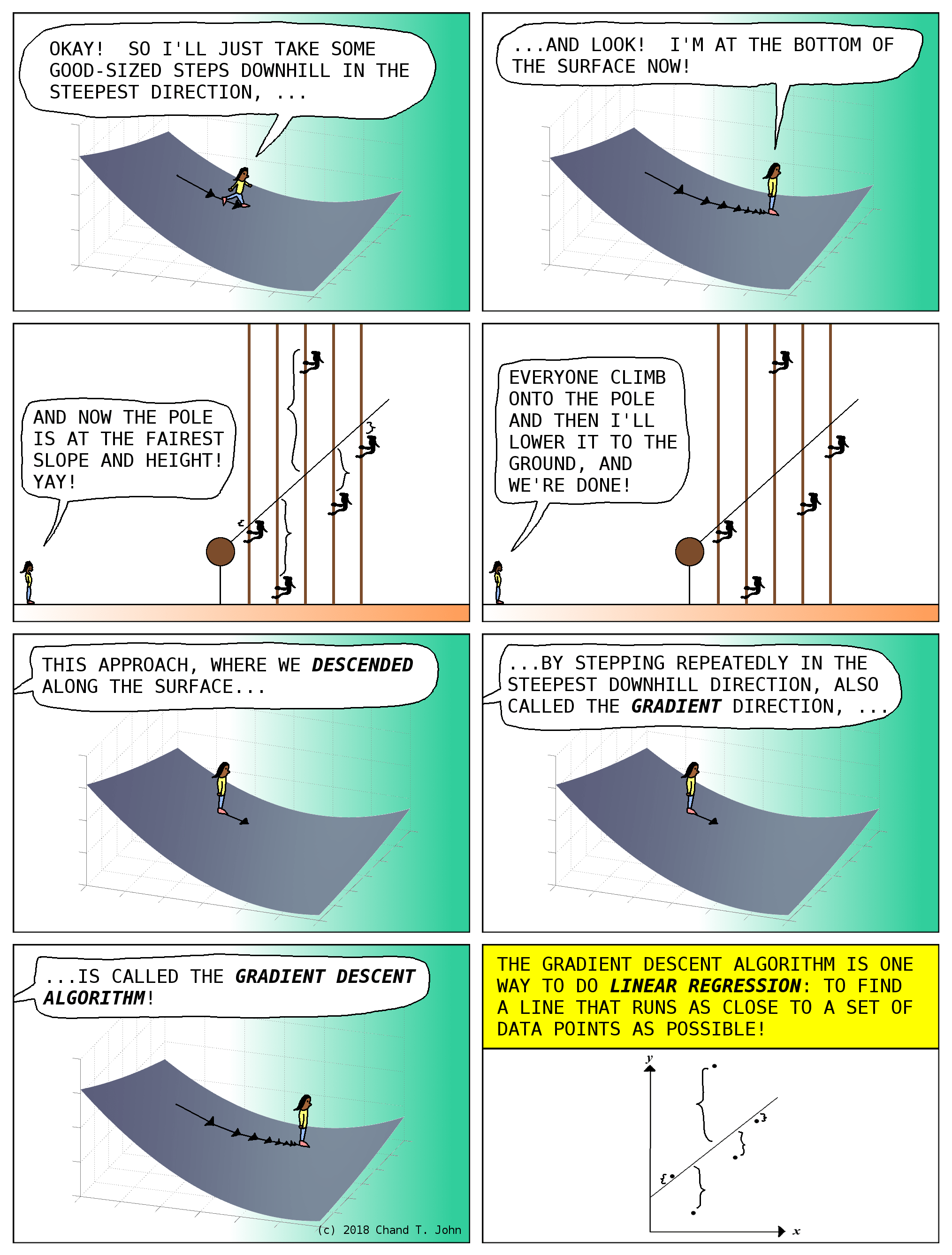In fact, there’s a faster way to jump to the lowest point of the surface in one step, using something called a Normal Equation.  This approach is described very clearly in Lesson 4 of Andrew Ng’s Coursera course on machine learning.  For the problem posed here, first, stack the horizontal positions of the five people, in meters, next to a column of five ones, into something called a matrix:

```[ 1 1 ]
[ 1 2 ]
[ 1 3 ]
[ 1 4 ]
[ 1 5 ]```

Second, rotate this matrix sideways into its transpose matrix:

```[ 1 1 1 1 1 ]
[ 1 2 3 4 5 ]```

Third, multiply this transpose matrix by the original matrix using an operation called matrix multiplication:

```             [ 1 1 ]
[ 1 2 ]
[ 1 1 1 1 1 ][ 1 3 ] = [  5 15 ]
[ 1 2 3 4 5 ][ 1 4 ]   [ 15 55 ]
[ 1 5 ]```

Fourth, calculate the matrix inverse of the resulting matrix:

```[  5 15 ]^-1   [  1.1  -0.3 ]
[       ]    = [            ]
[ 15 55 ]      [ -0.3   0.1 ]```

Fifth, multiply the resulting matrix again by the transpose matrix above:

```[  1.1  -0.3 ][ 1 1 1 1 1 ]   [  0.8  0.5     0.2 -0.1 -0.4 ]
[            ][           ] = [                             ]
[ -0.3   0.1 ][ 1 2 3 4 5 ]   [ -0.2 -0.1 -0.5511  0.1  0.2 ]```

Sixth, stack the height each person climbed to, in meters, into another matrix called a column vector:

```[ 3 ]
[ 1 ]
[ 9 ]
[ 4 ]
[ 6 ]```

Last, multiply last matrix we just calculated, by this column vector:

```                               [ 3 ]
[  0.8  0.5     0.2 -0.1 -0.4 ][ 1 ]   [ 1.9 ]
[                             ][ 9 ] = [     ]
[ -0.2 -0.1 -0.5511  0.1  0.2 ][ 4 ]   [ 0.9 ]
[ 6 ]```

These last two numbers give us the slope (0.9) and base height (1.9 meters) of the pole that would make the pole come as close to all five people as possible.

In terms of machine learning, we would say that the line that best fits the points (1, 3), (2, 1), (3, 9), (4, 4), and (5, 6), is the line that is the graph of the equation y = (0.9)x + 1.9.